1.1 最速下降法的原理

，我们想求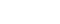。虽然下降方向有无穷多个，但是根据Cauchy-Schwarz不等式：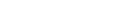当且仅当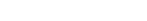时等式成立，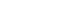## 1.2 最速下降法的收敛速度

#### 1.2.1  收敛性

1.2.2  预备知识Kantorovich不等式：#### 1.2.3  收敛速度的上界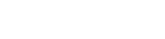1.2.4  收敛速度的差异性来源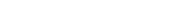,正定二次函数：的导函数方程相应变成了
，方程的解记为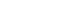,其中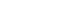非奇异，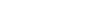满足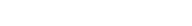非零。那幺：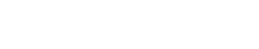1.2.5  最速下降法的优缺点

：算法每次迭代的计算量少，储存量也少，从一个不太好的初始点出发也能靠近极小点。

Zigzag现象（收敛慢的原因）：若迭代步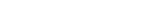的精确最小点，则
，因此：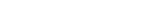，也就是上一步的方向与下一步的方向垂直。#### 2.1 基本Newton方法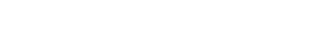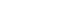。在点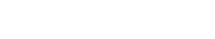去近似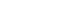，求解问题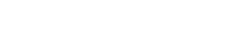。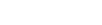为问题的唯一解。我们称为Newton方向。(Hesse的逆矩阵度量下的最速下降法)（1）当初始点接近极小点时，迭代序列收敛于极小点，并且收敛很快（二阶收敛）；

（2）当初始点不接近极小点时，迭代序列容易收敛到鞍点或者极大点（局部收敛性而不是全局收敛）。

（3）迭代过程可能会出现奇异矩阵或者病态，以至于求逆很困难，导致迭代失败。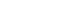的特征值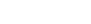求不出来。的特征值

（4）每一步迭代需要计算Hesse矩阵，即计算n(n+1)/2个二阶偏导数，相当于求解一个线性方程组，计算量为O(
)

## 2.2 阻尼Newton方法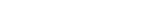。（对严格凸函数具有全局收敛性）

## 2.3 混合方法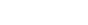。## 2.4 LM方法

LM方法是处理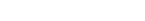来确定迭代方向的Newton型方法，这里的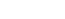是单位阵。显然，若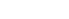正定。

（1）

（2）当不正定时，可以简单取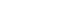Newton方法的优缺点：

（1）当初始点接近极小点时，迭代序列收敛于极小点，并且收敛很快（二阶收敛）；

（2）当初始点不接近极小点时，迭代序列容易收敛到鞍点或者极大点（局部收敛性而不是全局收敛）。

（3）迭代过程可能会出现奇异矩阵或者病态，以至于求逆很困难，导致迭代失败。的特征值求不出来。的特征值
,

（4）每一步迭代需要计算Hesse矩阵，即计算n(n+1)/2个二阶偏导数，相当于求解一个线性方程组，计算量为O(
)

## 3.1 拟牛顿条件

，已知条件为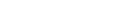，我们使用拉格朗日中值定理：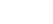得到
n个方程，n(n+1)/2个变量。，即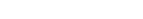，而

## 3.2.1 对称秩1公式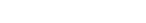代入拟牛顿方程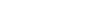得到：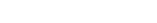。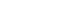共线，从而存在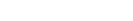。代入得到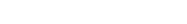,由此得到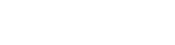.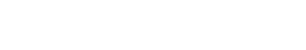，则需要用到SMW公式：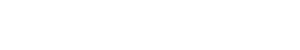## 3.2.2 对称秩2公式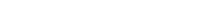，其中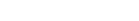待定。代入中，得到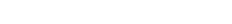## （1）DFP方法中，化简为，得到DFP公式：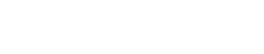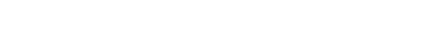## （2）BFGS公式（对偶）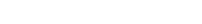用相同的推断实现：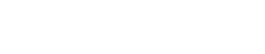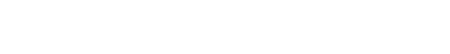## （3）Broyden族公式

DFP方法与BFGS公式的线性组合：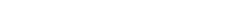## 3.3 三种拟牛顿方法的对比试验

#### （1）扩展Rosenbrock问题

(BFGS与DFP差异不大，SR1差些)（迭代次数与函数调用次数）（2）由人工神经网络解微分方程的问题：Rosenbrock函数的数据探索：````import numpy as np`
`import pandas as pd`
`import matplotlib.pyplot as plt`
`import time`
`%matplotlib inline`
`from mpl_toolkits.mplot3d import Axes3D`
`class Rosenbrock():`
`    def __init__(self):`
`        self.x1 = np.arange(-100, 100, 0.0001)`
`        self.x2 = np.arange(-100, 100, 0.0001)`
`        #self.x1, self.x2 = np.meshgrid(self.x1, self.x2)`
`        self.a = 1`
`        self.b = 1`
`        self.newton_times = 1000`
`        self.answers = []`
`        self.min_answer_z = []`
```
```
```
```
`    # 准备数据`
`    def data(self):`
`        z = np.square(self.a - self.x1) + self.b * np.square(self.x2 - np.square(self.x1))`
`        #print(z.shape)`
`        return z`
```
```
`    # 随机牛顿`
`    def snt(self,x1,x2,z,alpha):`
`        rand_init = np.random.randint(0,z.shape)`
`        x1_init,x2_init,z_init = x1[rand_init],x2[rand_init],z[rand_init]`
`        x_0 =np.array([x1_init,x2_init]).reshape((-1,1))`
`        #print(x_0)`
```
```
```
```
`        for i in range(self.newton_times):`
`            x_i = x_0 - np.matmul(np.linalg.inv(np.array([[12*x2_init**2-4*x2_init+2,-4*x1_init],[-4*x1_init,2]])),np.array([4*x1_init**3-4*x1_init*x2_init+2*x1_init-2,-2*x1_init**2+2*x2_init]).reshape((-1,1)))`
`            x_0 = x_i`
`            x1_init = x_0[0,0]`
`            x2_init = x_0[1,0]`
`        answer = x_0`
`        return answer`
```
```
```
```
`    # 绘图`
`    def plot_data(self,min_x1,min_x2,min_z):`
`        x1 = np.arange(-100, 100, 0.1)`
`        x2 = np.arange(-100, 100, 0.1)`
`        x1, x2 = np.meshgrid(x1, x2)`
`        a = 1`
`        b = 1`
`        z = np.square(a - x1) + b * np.square(x2 - np.square(x1))`
`        fig4 = plt.figure()`
`        ax4 = plt.axes(projection='3d')`
`        ax4.plot_surface(x1, x2, z, alpha=0.3, cmap='winter')  # 生成表面， alpha 用于控制透明度`
`        ax4.contour(x1, x2, z, zdir='z', offset=-3, cmap="rainbow")  # 生成z方向投影，投到x-y平面`
`        ax4.contour(x1, x2, z, zdir='x', offset=-6, cmap="rainbow")  # 生成x方向投影，投到y-z平面`
`        ax4.contour(x1, x2, z, zdir='y', offset=6, cmap="rainbow")  # 生成y方向投影，投到x-z平面`
`        ax4.contourf(x1, x2, z, zdir='y', offset=6, cmap="rainbow")  # 生成y方向投影填充，投到x-z平面，contourf()函数`
`        ax4.scatter(min_x1,min_x2,min_z,c='r')`
`        # 设定显示范围`
`        ax4.set_xlabel('X')`
`        ax4.set_ylabel('Y')`
`        ax4.set_zlabel('Z')`
`        plt.show()`
```
```
`    # 开始`
`    def start(self):`
`        times = int(input("请输入需要随机优化的次数："))`
`        alpha = float(input("请输入随机优化的步长"))`
`        z = self.data()`
`        start_time = time.time()`
`        for i in range(times):`
`            answer = self.snt(self.x1,self.x2,z,alpha)`
`            self.answers.append(answer)`
`        min_answer = np.array(self.answers)`
`        for i in range(times):`
`            self.min_answer_z.append((1-min_answer[i,0,0])**2+(min_answer[i,1,0]-min_answer[i,0,0]**2)**2)`
`        optimal_z = np.min(np.array(self.min_answer_z))`
`        optimal_z_index = np.argmin(np.array(self.min_answer_z))`
`        optimal_x1,optimal_x2 = min_answer[optimal_z_index,0,0],min_answer[optimal_z_index,1,0]`
`        end_time = time.time()`
`        running_time = end_time-start_time`
`        print("优化的时间:%.2f秒!" % running_time)`
`        self.plot_data(optimal_x1,optimal_x2,optimal_z)`
`if __name__ == '__main__':`
`    snt = Rosenbrock()`
`    snt.start()````

`请输入需要随机优化的次数：100`1. 《Newton vs the machine: solving the chaotic three-body problem using deep neural networks》P G. Breen, C N. Foley, T Boekholt, S P Zwart

2.《Quasi-Newton Optimization Methods For Deep Learning Applications》J Rafati, R F. Marcia